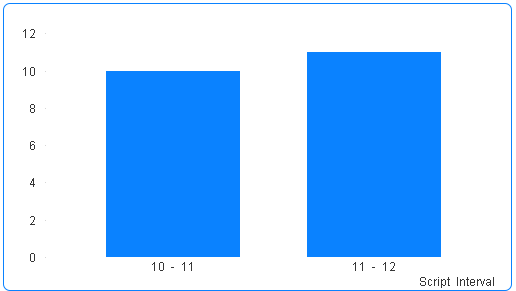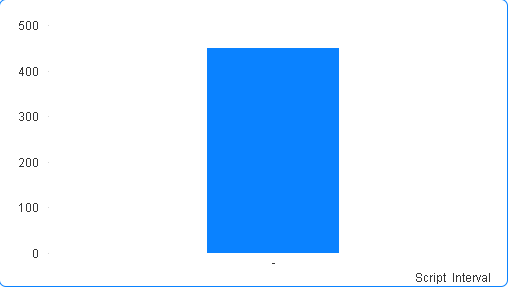QlikView App Dev

Discussion Board for collaboration related to QlikView App Development.

Announcements
QlikWorld 2022, LIVE in Denver CO., May 16-19, 2022. REGISTER NOW TO RECEIVE EARLY BIRD PRICING
cancel
Showing results for
Did you mean:Specialist

Class Function

I need to create a bar chart using the class function.  I have the following data:With the above data, I need to create a bar chart with a class interval of 1 on the X axis denoting the number of items per prescription interval, which will be count(Distinct [Script Number] ) and the number of Doctors which should be count(Distinct [Doctor Name]) on the Y axis.  How can i achieve this with the Class function?

Regards

Chris

1 Solution

Accepted SolutionsSpecialist
Author

Hi Yann

Many thanks, i have changed the dimension along your first suggestions to the following:

=Replace(Aggr(Class(count(DISTINCT [Drug Name]),1), [Script Number]), '<= x <', ' - ')

The expression is as follows:

=Aggr(count([Doctor Name]), [Script Number])

The result is as follows:This more or less what I am expecting.  The only problem is that I do not see any classes for Script Intervals less than 10.  Would you suggest any solution?

6 RepliesNot applicable

Hi,

I'm not sure of what you want exactly, but I think it should be something like this :

dimension : =class(Aggr(count([Drug Name]),[Script number]),1)  (or even directly =Aggr(count([Drug Name]),[Script number]) if the class interval is 1)

expression : count(distinct [Script number])

I hope it helps

YannSpecialist
Author

Hi Yann

Many thanks, i get the following results after changing the expression to Count(Distinct [Doctor Name]):Either of the two dimensions yields the same result.  This is showing the count of all doctors on the  Y axis but i need this to be the count of doctors for a specific script interval.  I hope this clarifies what i am looking for.

Thank you.Not applicable

So you should use this as dimension  :

=class([Script Number],1000, 'xxxx')Specialist
Author

Hi Yann

Many thanks, i have changed the dimension along your first suggestions to the following:

=Replace(Aggr(Class(count(DISTINCT [Drug Name]),1), [Script Number]), '<= x <', ' - ')

The expression is as follows:

=Aggr(count([Doctor Name]), [Script Number])

The result is as follows:This more or less what I am expecting.  The only problem is that I do not see any classes for Script Intervals less than 10.  Would you suggest any solution?MVP

as Count() delivers integer values, using the class function with an interval of 1 might be less readable than just displaying the integer itself, so:

=Aggr(count(DISTINCT [Drug Name]), [Script Number])

might actually be enough.

hope this helps

regards

MarcoSpecialist
Author

Many thanks MarcoCommunity Browser